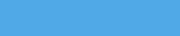# Bluish Coder

Programming Languages, Martials Arts and Computers. The Weblog of Chris Double.

2011-10-19

ATS allows overloading of functions where the function that is called is selected based on number of arguments and the type of the arguments. The following example shows overloading based on type to provide a generic print function:

``````symintr myprint
fun myprint_integer (a: int)    = printf("%d\n", @(a))
fun myprint_double  (a: double) = printf("%f\n", @(a))
fun myprint_string  (a: string) = printf("%s\n", @(a))

implement main() = {
val () = myprint ("hello")
val () = myprint (10)
val () = myprint (20.0)
}
``````

The keyword `symintr` introduces a symbol that can be overloaded. The keyword `overload` will overload that symbol with an existing function. In this example we overload with three functions that take different types. The overload resolution is performed at compile time. The actual C code generated for the main function includes:

``````/* tmp4 = */ myprint_string_2 (ATSstrcst("hello")) ;
/* tmp5 = */ myprint_integer_0 (10) ;
/* tmp3 = */ myprint_double_1 (20.0) ;
``````

The ATS standard prelude includes a `print` function that is overloaded in this manner for most of the standard types. One downside to the way overloading works is the overload resolution sometimes fails in template functions. The following code gives a compile error for example:

``````fun {a:t@ype} printme (x: a) = print(x)

implement main() = {
val () = printme (10)
val () = printme (20.0)
}
``````

The error given is that the symbol `print` cannot be resolved. The ATS compiler attempts to resolve the overload by looking up the `t@ype` sort. There is no overload for this so the resolution fails. This can be worked around using a template function to call the overloaded function and partially specialize the implementation of the new template function. The following code demonstrates this:

``````extern fun {a:t@ype} gprint (x: a):void
implement gprint<int> (x) = print_int(x)
implement gprint<double> (x) = print_double(x)

fun {a:t@ype} printme (x: a):void = gprint<a>(x)

implement main() = {
val () = printme (10)
val () = printme (20.0)
}
``````

The `print` symbol can be overloaded with the new `gprint` function to allow `print` to be called over `t@ype` sorts:

``````extern fun {a:t@ype} gprint (x: a):void
implement gprint<int> (x) = print_int(x)
implement gprint<double> (x) = print_double(x)

fun {a:t@ype} printme (x: a) = print(x)

implement main() = {
val () = printme (10)
val () = printme (20.0)
}
``````

This example is contrived in that you could just specialize `printme` but in real world code this issue comes up occasionally. The most common example for me has been using `=` in a template function, comparing arguments that are template type parameters. `=` is an overloaded function and the overload lookup fails in the same manner as above. A workaround is to create an `equals` template function specialized over the types you plan to compare as above.

### Tags

This site is accessable over tor as hidden service 6vp5u25g4izec5c37wv52skvecikld6kysvsivnl6sdg6q7wy25lixad.onion, or Freenet using key:
USK@1ORdIvjL2H1bZblJcP8hu2LjjKtVB-rVzp8mLty~5N4,8hL85otZBbq0geDsSKkBK4sKESL2SrNVecFZz9NxGVQ,AQACAAE/bluishcoder/-61/

Tags

Archives